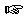### Section 12

Problem 1:

Develop the function sort-auction-records-by-bid. The function consumes a list of auction records and returns a list of auction records sorted in decreasing order by bid amount.

An auction record is defined as follows:

`(define-struct auction (item id bid))`

An auction record is a structure
`   (make-auction String Number Number)`Solution

Problem 2:
Develop the function cancel-sum . The function consumes a list of numbers. The function should produce a new list of numbers one longer in length than the original list. The new list should be the same as the old with with an additional number at the end that will cause the sum of the numbers in the new list to be zero.Solution

Problem 3:
Develop the function search-lolos. The function consumes a symbol and a list of list of symbols and returns true if the symbol is found anywhere in the original list of lists; otherwise, it returns false.Solution

Problem 4:
Develop the function expand-lon. The function consumes a list of natural numbers and returns a new list of natural numbers with each number in the original list replaced by a number of copies of that number equal to its value. For example, the function called with the list containing one and two would produce the list containing one, two, and two.Solution

Problem 5:
Develop the function complete-polygon. The function consumes a list of posns and a symbol representing a color and draws lines from every posn to every other posn. Be sure to load the draw teachpack before testing.Solution

 Jamie Raymond Matthias Felleisen 01 december 2003